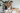# Using Multiples of 8 To Know What Least Common Multiple Means

POSTED ON APRIL 06, 2023The least common multiple shows the first and the smallest multiple shared between two or more numbers. It’s time to extend your knowledge of multiples to finding common multiples between two or more numbers!

## What Is a Least Common Multiple and How Do We Use Multiples of 8 To Understand LCM?

The least common multiple (also known as the LCM) represents the smallest number that is a multiple shared in common between two or more number. Before diving into the main topic, let’s take a quick refresher on what multiples are: a series or list of numbers that are the result of multiplying one number by other natural numbers.For example, the multiples of 8 would be a list of numbers that are the result of multiplying 8 by each other natural number.Yes, these are the series of numbers you’ll see in a multiplication table for 8! This is why you can also find the multiples of 8 (or any number) by continuously adding up 8 to the succeeding number as shown above.

Curriculum created to bring out the best in every learner
Learn more about how Juni's curriculum was created and what unique features are included, like the Juni recording studio and code editor.

## Finding the Least Common Multiple by Listing Down Multiples

One way of finding the LCM of two or more numbers is by writing down each of the numbers’ multiples and then finding the first match. Remember, the least common multiple is also known as the lowest common multiple for a reason. The goal is to look for the smallest multiple that the numbers share.

1. List down the first few multiples of the smaller number.
2. Do the same for the bigger number until you spot a common multiple.
3. Continue this process if there are more than two numbers.
4. The smallest multiple shared between the numbers is what we call the LCM.For example, to find the LCM of 4 and 8, list down the multiples of 4 and 8 then look for common multiples shared between the two.Write down a few multiples of 4 and 8 then the first match– this represents the least common multiple shared by 4 and 8.While 4 and 8 share a lot of common multiples, LCM represents the smallest from this list of common multiples. This means that the least common multiple of 4 and 8 is 8.

Online Tutoring with Juni Tutor Bot - Get Free Help 24/7
Juni Tutor Bot is your friendly online tutoring assistant. Get immediate, free homework help and exam support for general math, calculus, algebra tutoring, and standardized test prep like SATs. Learn with a community of peers and tutors, supported by AI.

#### Example 1

Find the least common multiple of the following group of numbers.

a. 12 and 15

First, list down the common multiples of 12 and do the same for 15. Find the first match and that’s the LCM that we need!The first common multiple shared between 12 and 15 is 60, so the LCM of 12 and 15 is equal to 60.

b. 2, 5, and 8

When finding the LCM of more than two numbers, just do the same this down we’re working on more groups of multiples. Find the common multiple shared between the three multiples. Yes, all three must have that multiple!All three numbers have 40 as a common multiple, so the LCM of 2, 5, and 8 is 40.